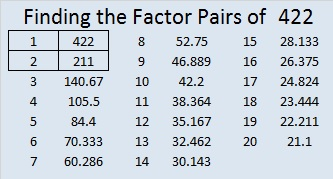# 422 and Level 3

422 has three even digits. How many factors could it possibly have? Scroll down to see.

This Find the Factors puzzle has the same color scheme that puzzles 414 and 417 had, but this one also has a couple of numbers that aren’t colored at all.  If 422 were colored, it would be yellow. I’ve had one very close guess posted in the comments for 417. Will anybody be able to read my mind and figure out what the coloring is all about? You can type your guess in the comments. Only an elementary education is required to figure it out!Print the puzzles or type the factors on this excel file: 12 Factors 2015-03-09

• 422 is a composite number.
• Prime factorization: 422 = 2 x 211
• The exponents in the prime factorization are 1 and 1. Adding one to each and multiplying we get (1 + 1)(1 + 1) = 2 x 2 = 4. Therefore 422 has exactly 4 factors.
• Factors of 422: 1, 2, 211, 422
• Factor pairs: 422 = 1 x 422 or 2 x 211
• 422 has no square factors that allow its square root to be simplified. √422 ≈ 20.5426A Logical Approach to FIND THE FACTORS: Find the column or row with two clues and find their common factor. Write the corresponding factors in the factor column (1st column) and factor row (top row).  Because this is a level three puzzle, you have now written a factor at the top of the factor column. Continue to work from the top of the factor column to the bottom, finding factors and filling in the factor column and the factor row one cell at a time as you go.